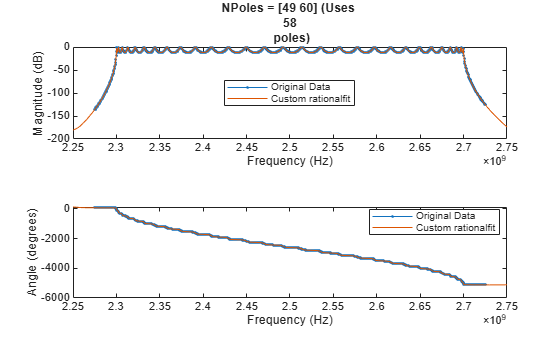# Using 'NPoles' Parameter With rationalfit

This example shows how to use the 'NPoles' parameter to improve the quality of the output of `rationalfit`. By default, the `rationalfit` function uses 48 or fewer poles to find the rational function that best matches the data. If 48 poles is not enough, it may be advantageous to change the range of the number of poles used by `rationalfit`.

First, read in the bandpass filter data contained in the file `npoles_bandpass_example.s2p`, and plot the `S21` data. Next, use the `rationalfit` function to fit a rational function to the `S21` data, with the 'NPoles' parameter set to its default value, and visually compare the results to the original data. Lastly, use `rationalfit` again, this time specifying a larger number of poles, and see if the result improves.

```S = sparameters('npoles_bandpass_example.s2p'); figure subplot(2,1,1) rfplot(S,2,1,'db') subplot(2,1,2) rfplot(S,2,1,'angle')```### Analyze Output of rationalfit When Using Default Value for 'NPoles'

Use the `rfparam` function to extract the `S21` values, and then call `rationalfit`.

```s21 = rfparam(S,2,1); datafreq = S.Frequencies; defaultfit = rationalfit(datafreq,s21);```
```Warning: Achieved only -13.0 dB accuracy with 48 poles, not -40.0 dB. Consider specifying a larger number of poles using the 'NPoles' parameter. ```

Use the `freqresp` function to calculate the response of the output of `rationalfit`.

```respfreq = 2.25e9:2e5:2.75e9; defaultresp = freqresp(defaultfit,respfreq);```

Compare the original data against the frequency response of the default rational function calculated by `rationalfit`.

```subplot(2,1,1) plot(datafreq,20*log10(abs(s21)),'.-') hold on plot(respfreq,20*log10(abs(defaultresp))) hold off xlabel('Frequency (Hz)') ylabel('Magnitude (dB)') defaultnpoles = numel(defaultfit.A); defaultstr = ['Default NPoles (Uses ',num2str(defaultnpoles),' poles)']; title(defaultstr) legend('Original Data','Default rationalfit','Location','best') subplot(2,1,2) plot(datafreq,unwrap(angle(s21))*180/pi,'.-') hold on plot(respfreq,unwrap(angle(defaultresp))*180/pi) hold off xlabel('Frequency (Hz)') ylabel('Angle (degrees)') legend('Original Data','Default rationalfit','Location','best')```Analyzing how well the output of `rationalfit` matches the original data, it appears that while the default values of `rationalfit` do a reasonably good job in the center of the bandpass region, the fit is poor on the edges of the bandpass region. It is possible that using a more complex rational function will achieve a better fit.

### Analyze Output of rationalfit When Using Custom Value for 'NPoles'

Fit the original `S21` data, but this time, instruct `rationalfit` to use between 49 and 60 poles using the 'NPoles' parameter.

```customfit = rationalfit(datafreq,s21,'NPoles',[49 60]); customresp = freqresp(customfit,respfreq);```

Compare the original data against the frequency response of the custom rational function calculated by `rationalfit`.

```figure subplot(2,1,1) plot(datafreq,20*log10(abs(s21)),'.-') hold on plot(respfreq,20*log10(abs(customresp))) hold off xlabel('Frequency (Hz)') ylabel('Magnitude (dB)') customnpoles = numel(customfit.A); customstr = ['NPoles = [49 60] (Uses ',num2str(customnpoles),' poles)']; title(customstr) legend('Original Data','Custom rationalfit','Location','best') subplot(2,1,2) plot(datafreq,unwrap(angle(s21))*180/pi,'.-') hold on plot(respfreq,unwrap(angle(customresp))*180/pi) hold off xlabel('Frequency (Hz)') ylabel('Angle (degrees)') legend('Original Data','Custom rationalfit','Location','best')```The fit using a larger number of poles is clearly more precise.